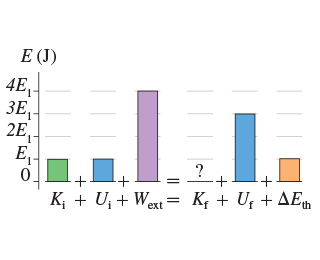Problem: Consider the process shown in the figure below. Suppose E1 = 3 J. What is the final kinetic energy of the system for the process shown in the figure?

FREE Expert Solution
100% (204 ratings)
Problem Details

Consider the process shown in the figure below. Suppose E1 = 3 J. What is the final kinetic energy of the system for the process shown in the figure?